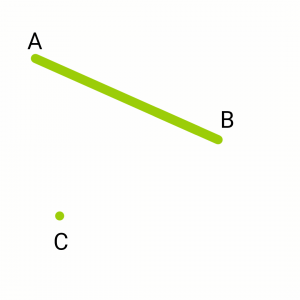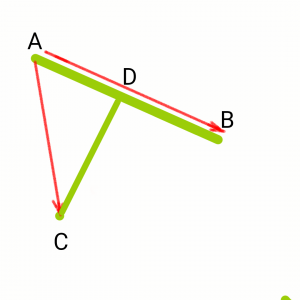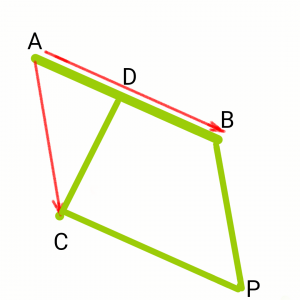# Shortest distance between a Line and a Point in a 3-D plane

Given a line passing through two points A and B and an arbitrary point C in a 3-D plane, the task is to find the shortest distance between the point C and the line passing through the points A and B.

Examples:

```Input: A = (5, 2, 1), B = (3, 1, -1), C = (0, 2, 3)
Output: Shortest Distance is 5

Input: A = (4, 2, 1), B = (3, 2, 1), C = (0, 2, 0)
Output: Shortest Distance is 1
```

Consider a point C and a line that passes through A and B as shown in the below figure.Now Consider the vectors, AB and AC and the shortest distance as CD. The Shortest Distance is always the perpendicular distance. The point D is taken on AB such that CD is perpendicular to AB.Construct BP and CP as shown in the figure to form a Parallelogram. Now C is a vertex of parallelogram ABPC and CD is perpendicular to Side AB. Hence CD is the height of the parallelogram.Note: In the case when D does not fall on line segment AB there will be a point D’ such that PD’ is perpendicular to AB and D’ lies on line segment AB with CD = PD’.

The magnitude of cross product AB and AC gives the Area of the parallelogram. Also, the area of a parallelogram is Base * Height = AB * CD. So,

`CD = |ABxAC| / |AB|`

Below is the CPP program to find the shortest distance:

 `// C++ program to find the Shortest ` `// Distance Between A line and a ` `// Given point. ` `#include ` `using` `namespace` `std; ` ` `  `class` `Vector { ` `private``: ` `    ``int` `x, y, z; ` `    ``// 3D Coordinates of the Vector ` ` `  `public``: ` `    ``Vector(``int` `x, ``int` `y, ``int` `z) ` `    ``{ ` `        ``// Constructor ` `        ``this``->x = x; ` `        ``this``->y = y; ` `        ``this``->z = z; ` `    ``} ` `    ``Vector operator+(Vector v); ``// ADD 2 Vectors ` `    ``Vector operator-(Vector v); ``// Subtraction ` `    ``int` `operator^(Vector v); ``// Dot Product ` `    ``Vector operator*(Vector v); ``// Cross Product ` `    ``float` `magnitude() ` `    ``{ ` `        ``return` `sqrt``(``pow``(x, 2) + ``pow``(y, 2) + ``pow``(z, 2)); ` `    ``} ` `    ``friend` `ostream& operator<<(ostream& out, ``const` `Vector& v); ` `    ``// To output the Vector ` `}; ` ` `  `// ADD 2 Vectors ` `Vector Vector::operator+(Vector v) ` `{ ` `    ``int` `x1, y1, z1; ` `    ``x1 = x + v.x; ` `    ``y1 = y + v.y; ` `    ``z1 = z + v.z; ` `    ``return` `Vector(x1, y1, z1); ` `} ` ` `  `// Subtract 2 vectors ` `Vector Vector::operator-(Vector v) ` `{ ` `    ``int` `x1, y1, z1; ` `    ``x1 = x - v.x; ` `    ``y1 = y - v.y; ` `    ``z1 = z - v.z; ` `    ``return` `Vector(x1, y1, z1); ` `} ` ` `  `// Dot product of 2 vectors ` `int` `Vector::operator^(Vector v) ` `{ ` `    ``int` `x1, y1, z1; ` `    ``x1 = x * v.x; ` `    ``y1 = y * v.y; ` `    ``z1 = z * v.z; ` `    ``return` `(x1 + y1 + z1); ` `} ` ` `  `// Cross product of 2 vectors ` `Vector Vector::operator*(Vector v) ` `{ ` `    ``int` `x1, y1, z1; ` `    ``x1 = y * v.z - z * v.y; ` `    ``y1 = z * v.x - x * v.z; ` `    ``z1 = x * v.y - y * v.x; ` `    ``return` `Vector(x1, y1, z1); ` `} ` ` `  `// Display Vector ` `ostream& operator<<(ostream& out, ` `                    ``const` `Vector& v) ` `{ ` `    ``out << v.x << ``"i "``; ` `    ``if` `(v.y >= 0) ` `        ``out << ``"+ "``; ` `    ``out << v.y << ``"j "``; ` `    ``if` `(v.z >= 0) ` `        ``out << ``"+ "``; ` `    ``out << v.z << ``"k"` `<< endl; ` `    ``return` `out; ` `} ` ` `  `// calculate shortest dist. from point to line ` `float` `shortDistance(Vector line_point1, Vector line_point2, ` `                    ``Vector point) ` `{ ` `    ``Vector AB = line_point2 - line_point1; ` `    ``Vector AC = point - line_point1; ` `    ``float` `area = Vector(AB * AC).magnitude(); ` `    ``float` `CD = area / AB.magnitude(); ` `    ``return` `CD; ` `} ` ` `  `// Driver program ` `int` `main() ` `{ ` `    ``// Taking point C as (2, 2, 2) ` `    ``// Line Passes through A(4, 2, 1) ` `    ``// and B(8, 4, 2). ` `    ``Vector line_point1(4, 2, 1), line_point2(8, 4, 2); ` `    ``Vector point(2, 2, 2); ` ` `  `    ``cout << ``"Shortest Distance is : "` `         ``<< shortDistance(line_point1, line_point2, point); ` ` `  `  ``return` `0; ` `} `

Output:

```Shortest Distance is : 1.63299
```

My Personal Notes arrow_drop_upCheck out this Author's contributed articles.

If you like GeeksforGeeks and would like to contribute, you can also write an article using contribute.geeksforgeeks.org or mail your article to contribute@geeksforgeeks.org. See your article appearing on the GeeksforGeeks main page and help other Geeks.

Please Improve this article if you find anything incorrect by clicking on the "Improve Article" button below.